# Creating a Pandas Series

Pandas Series is a one-dimensional labelled array capable of holding data of any type (integer, string, float, python objects, etc.). The axis labels are collectively called index.

Labels need not be unique but must be a hashable type. The object supports both integer and label-based indexing and provides a host of methods for performing operations involving the index.Creating an empty Series :
A basic series, which can be created is an Empty Series.

 `# import pandas as pd ` `import` `pandas as pd ` ` `  `# Creating empty series ` `ser ``=` `pd.Series() ` ` `  `print``(ser) `

Output :

```Series([], dtype: float64)
```

Creating a series from array:
In order to create a series from array, we have to import a numpy module and have to use array() function.

 `# import pandas as pd ` `import` `pandas as pd ` ` `  `# import numpy as np ` `import` `numpy as np ` ` `  `# simple array ` `data ``=` `np.array([``'g'``, ``'e'``, ``'e'``, ``'k'``, ``'s'``]) ` ` `  `ser ``=` `pd.Series(data) ` `print``(ser) `

Output :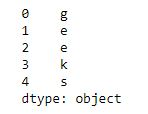Creating a series from array with index :
In order to create a series from array with index, we have to provide index with same number of element as it is in array.

 `# import pandas as pd ` `import` `pandas as pd ` ` `  `# import numpy as np ` `import` `numpy as np ` ` `  `# simple array ` `data ``=` `np.array([``'g'``, ``'e'``, ``'e'``, ``'k'``, ``'s'``]) ` ` `  `# providing an index ` `ser ``=` `pd.Series(data, index ``=``[``10``, ``11``, ``12``, ``13``, ``14``]) ` `print``(ser) `

Output :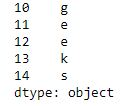Creating a series from Lists:
In order to create a series from list, we have to first create a list after that we can create a series from list.

 `import` `pandas as pd ` ` `  `# a simple list ` `list` `=` `[``'g'``, ``'e'``, ``'e'``, ``'k'``, ``'s'``] ` `  `  `# create series form a list ` `ser ``=` `pd.Series(``list``) ` `print``(ser) `

Output :Creating a series from Dictionary:
In order to create a series from dictionary, we have to first create a dictionary after that we can make a series using dictionary. Dictionary key are used to construct a index.

 `import` `pandas as pd ` `  `  `# a simple dictionary ` `dict` `=` `{``'Geeks'` `: ``10``, ` `        ``'for'` `: ``20``, ` `        ``'geeks'` `: ``30``} ` `  `  `# create series from dictionary ` `ser ``=` `pd.Series(``dict``) ` `  `  `print``(ser) `

Output :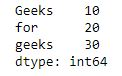Creating a series from Scalar value:
In order to create a series from scalar value, an index must be provided. The scalar value will be repeated to match the length of index.

 `import` `pandas as pd ` ` `  `import` `numpy as np ` ` `  `# giving a scalar value with index ` `ser ``=` `pd.Series(``10``, index ``=``[``0``, ``1``, ``2``, ``3``, ``4``, ``5``]) ` ` `  `print``(ser) `

Output :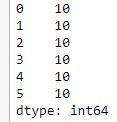Creating a series using NumPy functions :
In order to create a series using numpy function, we can use different function of numpy like numpy.linspace(), numpy.random.radn().

 `# import pandas and numpy  ` `import` `pandas as pd  ` `import` `numpy as np  ` `   `  `# series with numpy linspace()   ` `ser1 ``=` `pd.Series(np.linspace(``3``, ``33``, ``3``))  ` `print``(ser1)  ` `   `  `# series with numpy linspace()  ` `ser2 ``=` `pd.Series(np.linspace(``1``, ``100``, ``10``))  ` `print``(``"\n"``, ser2)  `

Output :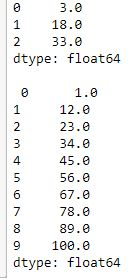My Personal Notes arrow_drop_upIf you like GeeksforGeeks and would like to contribute, you can also write an article using contribute.geeksforgeeks.org or mail your article to contribute@geeksforgeeks.org. See your article appearing on the GeeksforGeeks main page and help other Geeks.

Please Improve this article if you find anything incorrect by clicking on the "Improve Article" button below.

Article Tags :

2

Please write to us at contribute@geeksforgeeks.org to report any issue with the above content.